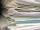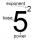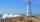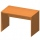# Examples for 8th grade - page 92

1. Hexagon cut pyramidCalculate the volume of a regular 6-sided cut pyramid if the bottom edge is 30 cm, the top edge us 12 cm, and the side edge length is 41 cm.
2. Three paintersThe three painters have painted bridge. The first would work done in 5 days, the second in 6 days, and the third in 7.5 days. How long will the bridge work if they work together?
3. PumpsAfter the floods, four equally powerful pumps exhausted water from the flooded cellar in 6 hours. How many hours would take a drained out with three equally powerful pumps?
4. Magic numberThe number 135 split to two addends so that one addend was 30 greater than 2/5 the addend.
5. Iron collectingClass 7A collected 3.2 tonnes of iron more than class 7B. Together they collected 6.4 tonnes of iron to the secondary raw material collection. How much did each class collect?
6. Surface of cubesPeter molded a cuboid 2 cm, 4cm, 9cm of plasticine. Then the plasticine split into two parts in a ratio 1:8. From each part made a cube. In what ratio are the surfaces of these cubes?
7. Area of iso-trapFind the area of an isosceles trapezoid, if the lengths of its bases are 16 cm, and 30 cm, and the diagonals are perpendicular to each other.
8. DiagonalsA diagonal of a rhombus is 20 cm long. If it's one side is 26 cm find the length of the other diagonal.
9. Diagonalhe rectangular ABCD trapeze, whose AD arm is perpendicular to the AB and CD bases, has area 15cm square. Bases have lengths AB = 6cm, CD = 4cm. Calculate the length of the AC diagonal.
10. Points on line segmentPoints P & Q belong to segment AB. If AB=a, AP = 2PQ = 2QB, find the distance: between point A and the midpoint of the segment QB.
11. RemainderA is an arbitrary integer that gives remainder 1 in the division with 6. B is an arbitrary integer that gives remainder 2 the division by. What makes remainder in division by 3 product of numbers A x B ?
12. MushroomsEva and Jane collected 114 mushrooms together. Eve found twice as much as Jane. How many mushrooms found each of them?
13. Trapezoid - intersection of diagonalsIn the ABCD trapezoid is AB = 8 cm long, trapezium height 6 cm, and distance of diagonals intersection from AB is 4 cm. Calculate trapezoid area.
14. EmbankmentPerpendicular cross-section of the embankment around the lake has the shape of an isosceles trapezoid. Calculate the perpendicular cross-section, where bank is 4 m high the upper width is 7 m and the legs are 10 m long.
15. Unknown number 17Milada said, I am thinking of a number such that I evaluate expression x1/3, the value of the expression would be 5. Which number Milada thinking?
16. CandyPeter had a sachet of candy. He wanted to share with his friends. If he gave them 30 candies, he would have 62 candies. If he gave them 40 candies, he would miss 8 candies. How many friends did Peter have?
17. How farFrom the top of a lighthouse 145 ft above sea level, the angle of depression of a boat 29°. How far is the boat from the lighthouse?
18. Unknown numbersThe sum of two consecutive natural numbers and their triple is 92. Find these numbers.
19. ClubhouseThere were only chairs and table in the clubhouse. Each chair had four legs, and the table was triple. Scouts came to the clubhouse. Everyone sat on their chair, two chairs were left unoccupied, and the number of legs in the room was 101. How many chairs w
20. Rhombus constructionConstruct ABCD rhombus if its diagonal AC=9 cm and side AB = 6 cm. Inscribe a circle in it touching all sides...

Do you have an interesting mathematical example that you can't solve it? Enter it, and we can try to solve it.

To this e-mail address, we will reply solution; solved examples are also published here. Please enter e-mail correctly and check whether you don't have a full mailbox.